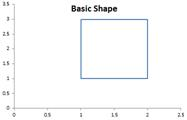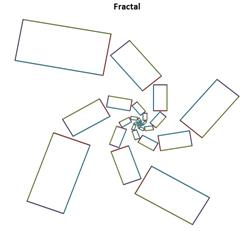## Fractal Geometry from Complex Number

In this section, we will generate a fractal geometry using iterated map. The procedure is so simple that you can even make it in a spreadsheet.

• Create basic shape and transform the coordinate of points to complex number• Apply Complex Linear Transformationfor any parametersandfor any parametersandand• Transformback to Real and Imaginary for plotting back to Real and Imaginary for plotting
• Use the previous results as input of transformation• Continue step 3 and 4 until say 100 steps
• Plot the Complex Plane and you will see fractal figures with self-similarity as zoom in and zoom out.

## Fractal Geometry Figures

The following fractal figures are made using a software. The original shape is on the top left (thick red). We use complex linear transformation with iterated map up to 1000 iteration and then we zoom in many times. You can see that as we zoom in, the shape remain the same and we will find out the same shape again and again. This characteristics is called self-similarity.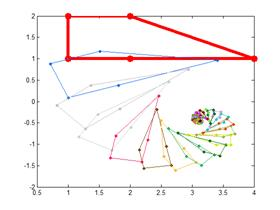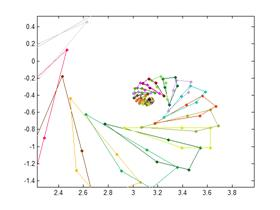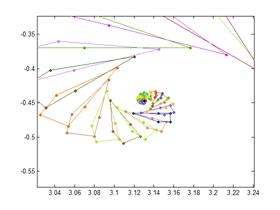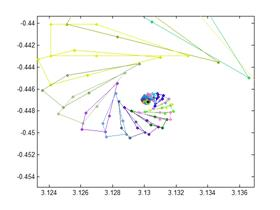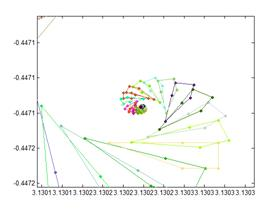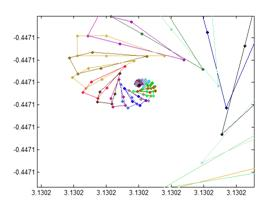Below are basic shape and fractal generated using Microsoft Excel.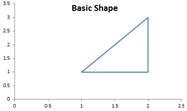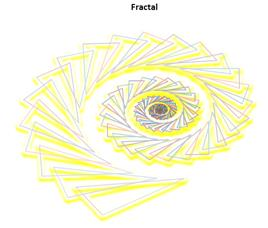If you change the basic shape and the parameters, you will obtain new fractal geometry